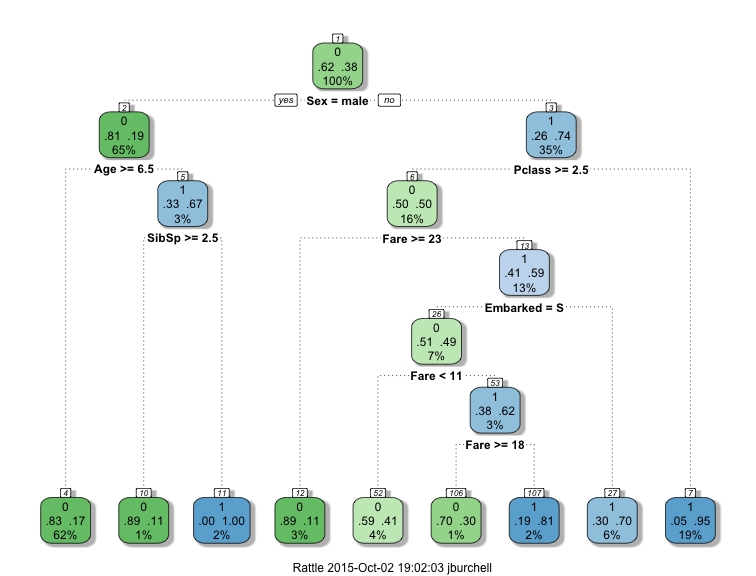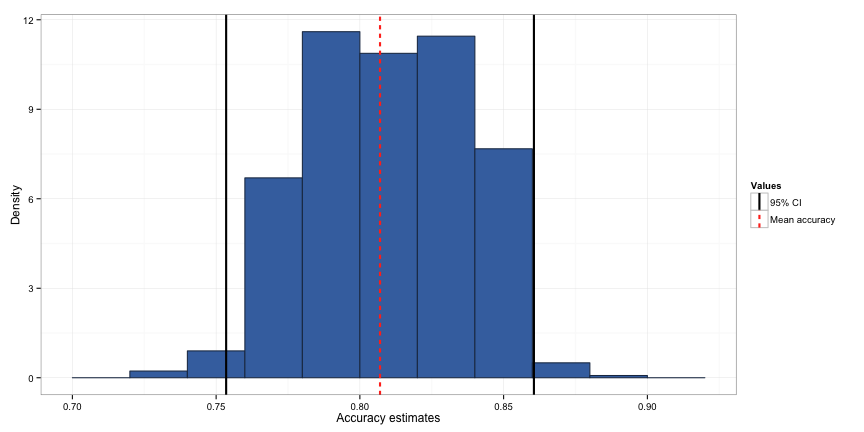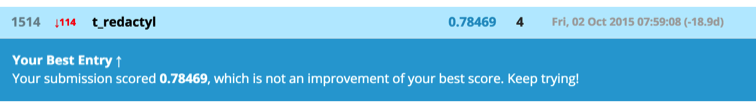# Using k-fold cross-validation to estimate out-of-sample accuracy

written in

One of the biggest issues when building an effective machine learning algorithm is overfitting. Overfitting is where you build a model on your training data and it not only picks up the true relationship between the outcome and the predictors, but also random noise specific to your training set. As such, the model will have much better performance on the training set than any new data, and will not generalise well outside the training set. In order to gauge the true predictive ability of our model on novel data, we need to build some way of estimating the out-of-sample accuracy into our model generation process. I’ll discuss one way of doing this, k-fold cross-validation, in this blog post, using the Kaggle Titanic tutorial dataset.

## Building a model

Firstly, we’ll load in our data:

train <- read.csv(url("http://s3.amazonaws.com/assets.datacamp.com/course/Kaggle/train.csv"))


and do a quick screening:

str(train)
table(train$Survived) prop.table(table(train$Survived))


We have 891 observations, with 549 (62%) people who died, and 342 (38%) people who survived.

Information on the variables in the Titantic dataset can be found here. For our model, we’ll use a decision-tree model with passenger class (“Pclass”), sex (“Sex”), age (“Age”), number of siblings or spouses aboard (“SibSp”), number of parents or children aboard (“Parch”), the passenger fare (“Fare”) and port of embarkation (C = Cherbourg; Q = Queenstown; S = Southampton) (“Embarked”). I used this model as it is one of those used in the excellent DataCamp R Titantic tutorial, so if you’re new to machine learning you can work your way through that to work out how we got to this point. You can see the model displayed in the figure below.

library(rpart); library(caret)
model.single <- rpart(Survived ~ Pclass + Sex + Age + SibSp + Parch + Fare + Embarked,
data = train, method = "class")
predict.single <- predict(object = model.single, newdata = train, type = "class")

library(RGtk2); library(cairoDevice); library(rattle); library(rpart.plot); library(RColorBrewer)
fancyRpartPlot(model.single)So how did our model perform on the data it was trained on?

confusionMatrix(predict.single, train$Survived)  ## Confusion Matrix and Statistics ## ## Reference ## Prediction 0 1 ## 0 521 115 ## 1 28 227 ## ## Accuracy : 0.8395 ## 95% CI : (0.8137, 0.863) ## No Information Rate : 0.6162 ## P-Value [Acc > NIR] : < 2e-16 ## ## Kappa : 0.6436 ## Mcnemar's Test P-Value : 6.4e-13 ## ## Sensitivity : 0.9490 ## Specificity : 0.6637 ## Pos Pred Value : 0.8192 ## Neg Pred Value : 0.8902 ## Prevalence : 0.6162 ## Detection Rate : 0.5847 ## Detection Prevalence : 0.7138 ## Balanced Accuracy : 0.8064 ## ## 'Positive' Class : 0 ##  We can see that our model has an accuracy of 0.84 when we apply it back to the data it was trained on. However, this is likely to be an overestimate. How do we get an idea of what the true accuracy rate would be on new data? ## Estimating out-of-sample accuracy In order to estimate the out-of-sample accuracy, we need to train the data on one dataset, and then apply it to a new dataset. The usual way to do this is to split the dataset into a training set and a testing set, build the model on the training set, and apply it to the testing set to get the accuracy of the model on new data. However, this method relies on having large datasets. In the case of smaller datasets (such as our Titanic data), an alternative to estimate out-of-sample accuracy is cross-validation. Cross-validation is kind of the same idea as creating single training and testing sets; however, because a single training and testing set would yield unstable estimates due to their limited number of observations, you create several testing and training sets using different parts of the data and average their estimates of model fit. ## k-fold cross-validation In k-fold cross-validation, we create the testing and training sets by splitting the data into $$k$$ equally sized subsets. We then treat a single subsample as the testing set, and the remaining data as the training set. We then run and test models on all $$k$$ datasets, and average the estimates. Let’s try it out with 5 folds: k.folds <- function(k) { folds <- createFolds(train$Survived, k = k, list = TRUE, returnTrain = TRUE)
for (i in 1:k) {
model <- rpart(Survived ~ Pclass + Sex + Age + SibSp + Parch + Fare + Embarked,
data = train[folds[[i]],], method = "class")
predictions <- predict(object = model, newdata = train[-folds[[i]],], type = "class")
accuracies.dt <- c(accuracies.dt,
confusionMatrix(predictions, train[-folds[[i]], ]$Survived)$overall[])
}
accuracies.dt
}

set.seed(567)
accuracies.dt <- c()
accuracies.dt <- k.folds(5)
accuracies.dt

##  0.8033708 0.7696629 0.7808989 0.8435754 0.8258427

mean.accuracies <- mean(accuracies.dt)


As you can see above, this function produces a vector containing the accuracy scores for each of the 5 cross-validations. If we take the mean and standard deviation of this vector, we get an estimate of our out-of-sample accuracy. In this case, this is estimated to be 0.805, which is quite a bit lower than our in-sample accuracy estimate.

## Repeated k-fold cross-validation

However, it is a bit dodgy taking a mean of 5 samples. On the other hand, splitting our sample into more than 5 folds would greatly reduce the stability of the estimates from each cross-validation. A way around this is to do repeated k-folds cross-validation. To do this, we simply repeat the k-folds cross-validation a large number of times and take the mean of this estimate. An advantage of this approach is that we can also get an estimate of the precision of this out-of-sample accuracy by creating a confidence interval. We’ll do 200 replications so we end up with a nice round 1,000 out-of-sample accuracy estimates.

set.seed(567)
v <- c()
v <- replicate(200, k.folds(5))
accuracies.dt <- c()
for (i in 1 : 200) {
accuracies.dt <- c(accuracies.dt, v[,i])
}

mean.accuracies <- mean(accuracies.dt)
lci <- mean(accuracies.dt) - sd(accuracies.dt) * 1.96
uci <- mean(accuracies.dt) + sd(accuracies.dt) * 1.96


This time, we get an estimate of 0.807, which is pretty close to our estimate from a single k-fold cross-validation. As you can see from our the histogram below, the distribution of our accuracy estimates is roughly normal, so we can say that the 95% confidence interval indicates that the true out-of-sample accuracy is likely between 0.753 and 0.861.## Testing out the model in Kaggle

Finally, let’s see how our out-of-sample accuracy estimate performs on the unlabelled Kaggle test set. First, let’s apply the model to the test set, then export a .csv file containing only the passenger ID and our prediction. We then submit this to Kaggle.

# Read in test data

# Apply the model to the test data
predict.test <- predict(object = model.single, newdata = test, type = "class")

# Create a data frame with just PassengerId and Survived to submit to Kaggle. Note that I assign "predict.test" to "Survived"
titanic_solution <- data.frame(PassengerId = test\$PassengerId, Survived = predict.test)

# Write your solution to a csv file with the name my_solution.csv
write.csv(titanic_solution, file = "titanic_solution.csv", row.names = FALSE)We end up with a final accuracy rating on Kaggle of 0.785, which is lower than our mean accuracy estimate, but within our 95% confidence interval. We can also see that it is substantially lower than the in-sample accuracy we got at the beginning of this post, indicating that the model is overfitting the training data.

## Take-away message

I hope this has been a helpful introduction to the importance of estimating the out-of-sample accuracy of your machine learning algorithm, and how to do so on smaller datasets. While it is preferrable to estimate out-of-sample accuracy on a new testing dataset, time and monetary constraints can limit how easily you can collect large labelled datasets. As such, cross-validation is an important tool in the data scientist’s toolkit.